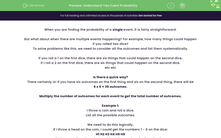# Understand Two Event Probability

In this worksheet, students will practise listing all the outcomes of multiple events and use them to find probabilities.Key stage:  KS 4

Year:  GCSE

GCSE Subjects:   Maths

GCSE Boards:   AQA, Eduqas, Pearson Edexcel, OCR,

Curriculum topic:   Probability

Curriculum subtopic:   Probability Basic Probability and Experiments

Popular topics:   Probability worksheets

Difficulty level:#### Worksheet Overview

When you are finding the probability of a single event, it is fairly straightforward.

But what about when there are multiple events happening? For example, how many things could happen if you rolled two dice?

To solve problems like this, we need to consider all the outcomes and list them systematically.

If you roll a 1 on the first dice, there are six things that could happen on the second dice.

If I roll a 2 on the first dice, there are six things that could happen on the second dice.

etc etc

Is there a quick way?

There certainly is! If you have six outcomes on the first thing and six on the second thing, there will be

6 x 6 = 36 outcomes.

Multiply the number of outcomes for each event to get the total number of outcomes.

Example 1:

I throw a coin and roll a dice.

List all the possible outcomes.

We need to do this logically.

If I throw a head on the coin, I could get the numbers 1 - 6 on the dice:

H1 H2 H3 H4 H5 H6

If I throw a tail on the coin, I could get the numbers 1 - 6 on the dice:

T1 T2 T3 T4 T5 T6

As a double-check, I have two outcomes on the coin and six on the dice. This means I should have

2 x 6 = 12 outcomes. (And I do!)

Example 2:

I throw a coin and roll a dice.

What is the probability that I get a head and an even number?

Our first step is to list all the outcomes:

H1 H2 H3 H4 H5 H6

T1 T2 T3 T4 T5 T6

We can now identify which ones satisfy our condition (head and even number):

H1 H2 H3 H4 H5 H6

T1 T2 T3 T4 T5 T6

We can see that there are twelve different outcomes in total and three outcomes that satisfy the condition: H2 H4 H6

This gives a probability of 3/12 which simplifies to 1/4

Now let's try some questions.

### What is EdPlace?

We're your National Curriculum aligned online education content provider helping each child succeed in English, maths and science from year 1 to GCSE. With an EdPlace account you’ll be able to track and measure progress, helping each child achieve their best. We build confidence and attainment by personalising each child’s learning at a level that suits them.

Get started